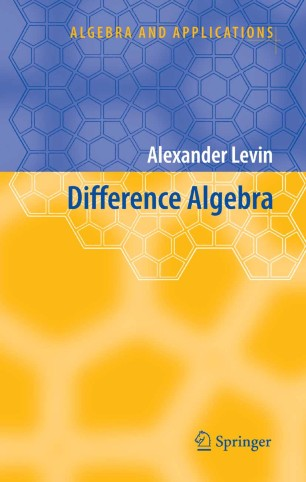# Difference Algebra

• First monograph on difference algebra that covers partial algebraic structures, and the only monograph on the subject published in the last forty years

• Contains new ideas and technique (such as construction of Gröbner bases with respect to several orderings and the concepts of multivariable dimension polynomials) that can be efficiently applied in various areas of algebra and algebraic geometry

• Contains an important application of the algebraic technique to the study of the A. Einstein's concept of strength of systems of difference equations of mathematical physicsBook

Part of the Algebra and Applications book series (AA, volume 8)

1. Front Matter
Pages i-xi
2. Pages 1-102
3. Pages 103-154
4. Pages 155-244
5. Pages 245-309
6. Pages 311-370
7. Pages 393-462
8. Pages 463-493
9. Back Matter
Pages 495-519

### Introduction

Difference algebra grew out of the study of algebraic difference equations with coefficients from functional fields in much the same way as the classical algebraic geometry arose from the study of polynomial equations with numerical coefficients. The first stage of the development of the theory is associated with its founder J. F. Ritt (1893 - 1951) and R. Cohn whose book Difference Algebra (1965) remained the only fundamental monograph on the subject for many years. Nowadays, difference algebra has overgrew the frame of the theory of ordinary algebraic difference equations and appears as a rich theory with applications to the study of equations in finite differences, functional equations, differential equations with delay, algebraic structures with operators, group and semigroup rings.

This book reflects the contemporary level of difference algebra; it contains a systematic study of partial difference algebraic structures and their applications, as well as the coverage of the classical theory of ordinary difference rings and field extensions. The monograph is intended for graduate students and researchers in difference and differential algebra, commutative algebra, ring theory, and algebraic geometry. It will be also of interest to researchers in computer algebra, theory of difference equations and equations of mathematical physics. The book is self-contained; it requires no prerequisites other than knowledge of basic algebraic concepts and mathematical maturity of an advanced undergraduate.

### Keywords

Algebra Algebraic difference equation Algebraic structure Difference field extension Difference module Difference polynomial Difference ring difference kernel ring theory

#### Authors and affiliations

1. 1.The Catholic University of AmericaWashington, D.C.USA

### Bibliographic information

• Book Title Difference Algebra
• Authors Alexander Levin
• Series Title Algebra and Applications
• DOI https://doi.org/10.1007/978-1-4020-6947-5
• Publisher Name Springer, Dordrecht
• eBook Packages Mathematics and Statistics Mathematics and Statistics (R0)
• Hardcover ISBN 978-1-4020-6946-8
• Softcover ISBN 978-90-481-7774-5
• eBook ISBN 978-1-4020-6947-5
• Edition Number 1
• Number of Pages XI, 521
• Number of Illustrations 0 b/w illustrations, 0 illustrations in colour
• Topics
• Buy this book on publisher's site

## Reviews

From the reviews:

“Levin’s Difference Algebra  is a milestone in the subject. It is an ever so fundamental and detailed work, in which one does not require the ordinary case of one selected automorphism…an excellent source of numerous results and techniques” (Bulletin of the London Mathematical Society, April 16, 2011)

“This book gives a systematic study of both ordinary and partial difference algebraic structures and their applications. … The book will long become a good reference for researchers in the area of difference algebra and algebraic structures with operators.” (Hirokazu Nishimura, Zentralblatt MATH, Vol. 1209, 2011)